# CSE1PES programming for engineers and scientists

BACKGROUND

An Alternating Current (AC) circuit is one in which the output of the voltage source changes with time. When we add components to the circuit, the voltage and current will be different at different points in the circuit. The components we will be looking at are resistors, capacitors and inductors. Further information can be found here: https://en.wikipedia.org/wiki/Alternating_current, https://en.wikipedia.org/wiki/Inductor, https://en.wikipedia.org/wiki/Resistor, https://en.wikipedia.org/wiki/Capacitor.

Impedance is the measure of the resistance and reactance of a component or circuit. Resistance is a measure of friction against the motion of electrons, whereas reactance is the inertia against the motion of electrons. Impedance introduces a phase shift between the voltage and current through a component, and hence is expressed as a complex number. Do not worry too much if this doesn't make sense, you can just think of this as complex resistance. For further information, please read https://en.wikipedia.org/wiki/Electrical_impedance.

To calculate the impedance, voltage and current for each component in the circuit, we can use ohms law. The equation for ohms law is as follows: (Note we are dealing with complex numbers, however we will develop all the necessary functions you will need in lab 5)

V = I*Z,I = V/Z, Z = V/I

Where V, I and Z represent voltage, current and impedance respectively, and are measured in volts, amps and ohms respectively.

For series circuits the current is the same through all components. For parallel circuits the voltage is the same through all components.

The impedance calculation depends on the component. Details are as follows:

 Resistors: The impedance for a resistor can be found as: 𝑍 = 𝑅 where R is the resistance measured in ohms. Capacitors: The impedance for a capacitor can be found as: 𝑋𝑐 = 1/2∗𝜋∗𝑓∗𝐶 where C is the capacitance measured in Farads and f is the frequency measured in hertz 𝑍 = 0 − 𝑗 ∗ 𝑋𝑐 where 𝑋𝑐 is the reactance and j is the imaginary unit. Inductors: The impedance for an inductor can be found as: 𝑋𝐿 = 2 ∗ 𝜋 ∗ 𝑓 ∗ 𝐿 where L is the inductance measured in Henrys 𝑍 = 0 + 𝑗 ∗ 𝑋𝐿 where 𝑋𝐿 is the reactance and j is the imaginary unit.

Components will either be arranged in parallel or series. Depending on this, the total impedance calculation will vary.

The total impedance between two components in series is:

𝑍𝑇 = 𝑍1 + 𝑍2

If we have three components in series, then the total impedance is:

𝑍𝑇2 = 𝑍𝑇 + 𝑍3

Hence, generalizing this we get:

𝑛

𝑍𝑇 = ∑ 𝑍𝑘

𝑘=1

The total impedance between two components in parallel is:

1 1 −1

𝑍𝑇 = (𝑍1 + 𝑍2)

Which we can then extend to calculate for three components by:

1 1 −1

𝑍𝑇2 = (𝑍𝑇 + 𝑍3)

Hence, generalizing this we get: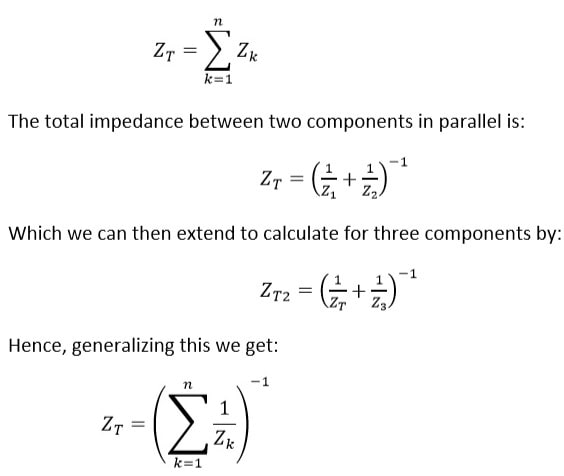### Problem

You are to create a program that allows a user to view the voltage, current and impedance for each component and the total in either the parallel circuit (a) or series circuit (b). The program will ask the user for the circuit type, the source voltage, the frequency, the number of components, and the values of each component. The two circuits are shown below: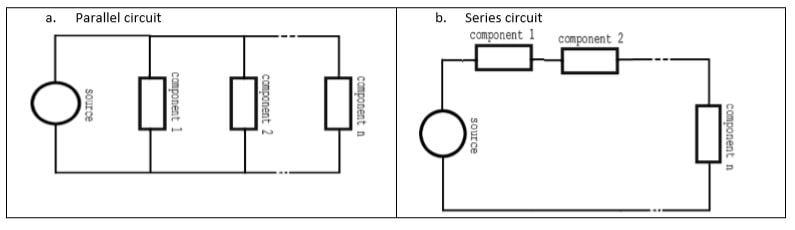Given a parallel circuit (a), with a source voltage of 12Vpp, a frequency of 60Hz, with 3 components in parallel. The components are a 40 ohm resistor, a 0.0003 farad capacitor and a 0.2 henry inductor.

Firstly we calculate the impedance of each component:

𝑍𝑅(𝑖𝑚𝑝𝑒𝑑𝑎𝑛𝑐𝑒 𝑜𝑓 𝑟𝑒𝑠𝑖𝑠𝑡𝑜𝑟) 𝑚𝑠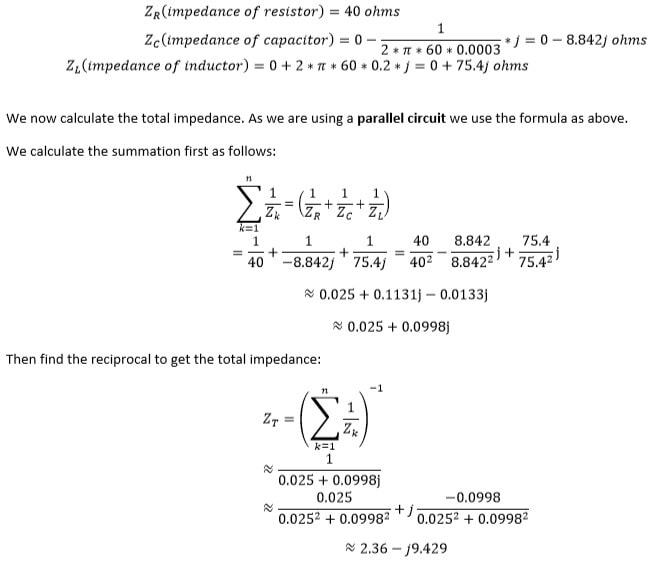We then calculate the total current using Ohms law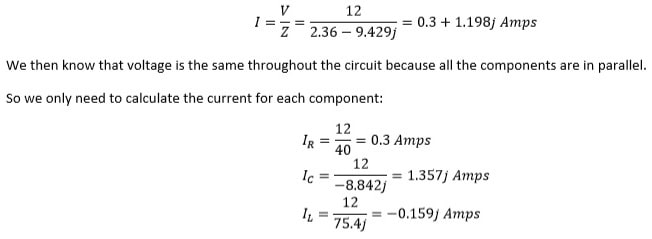Hence we can now generate the table:

 COMPONENTS R C L TOTAL Z ( Ohms) 40+0j 0-8.842j 0+75.4j 2.36 − 9.425𝑗 I (Amps) 0.3+0j 0+1.357j 0-0.159j 0.3+1.198j V (Volts) 12+0j 12+0j 12+0j 12+0j

FUNCTION PROTOTYPES AND INCLUDES

1. You must include the stdio.h and stdlib libraries
2. The following function prototypes are to be used: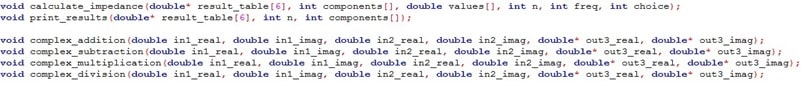1. The first two are described below
2. The last four will be developed in lab5, you need to alter the types from floats to doubles
3. You can change the naming
4. You cannot change the types

FUNCTION - MAIN

1. The program prints to the screen the student number, student name and the assignment number. This is enclosed by double asterisks. Ensure you follow the below format.

**3777777 Jhon Smith Assignment 2 **

2. The program asks the user which circuit they want to evaluate, the frequency in hertz, the source voltage and the number of components. And stores the results appropriately. All of these values are positive integers. When asking for the number of components, the type of circuit should be specified as shown below.
1. You can assume the user enters valid values for frequency, voltage and number of components.
2. You must handle the case where the user enters an incorrect value for the type of circuit and give a message to the user. (No example given, a single line for ‘invalid message’ to user ending with a newline. The message is up to you, but must be appropriate)
3. The program creates an array of pointers to doubles(hint: use [ ]), the array is of length 6
1. Then using a for loop dynamically allocate memory (malloc) for each of the pointers an array of size sizeof(double) *(number of components + 1)
2. This is the result table
3. The program stores the source voltage in the array as shown below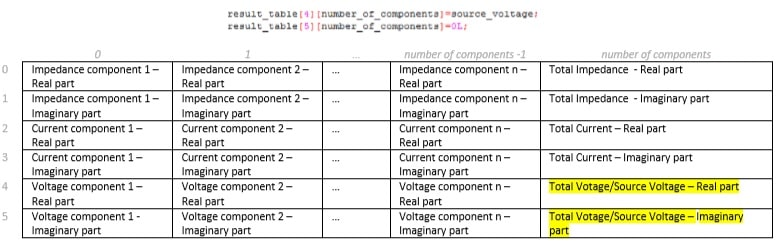1. The program creates a pointer to ints and dynamically allocates memory for an array of lengthnumber of components. This will store the component types.
2. The program creates a pointer to doubles and dynamically allocates memory for an array of lengthnumber of components. This will store the size of the components.
3. For each component, the program asks the user to enter the type of component and the value of that component. When asking for the size, the correct units must be shown (Ohms, Farads and Henrys).
1. The storing of the component types and values must be done using pointer arithmetic in this section. (Refer to lab 6 and additional material)
2. You can assume the user enters valid values components (1,2 or 3) and their values (positive values)
4. Calculate the impedance values by calling the calculate_impedance function. (Described in section below)
5. Calculate the total current values for both the real and imaginary parts and store appropriately (Ohms law I=V/Z)
6. Calculate the voltages and currents of all components, and store appropriately. (Hint. Refer to background information – Ohms Law. Parallel and series will have different equations)
7. Call the print_results function to print out the results table (Described in section below)
8. End the program appropriately.

FUNCTION - CALCULATE_IMPEDANCE

1. Calculate the impedance for both real and imaginary part for each component using a for loop with an if, else-if, else statement in the loop. And store the results in the results_table (Hint. Refer to background information – Impedance. Resistors, Capacitors and Inductors have different equations)
2. Calculate the total impedance for both real and Imaginary parts. And store the results in the results_table (Refer to additional material if new to summation)

Hint: (One possible implementation)

1. Create temporary variables to store the current sum (for both complex and real parts) and initialize to 0.
2. Create variables to store the total impedance (both for complex and real parts).
3. Then if the circuit is series:
1. Iteratively add to these for each impedance values.
2. The sum is the total impedance
4. else if the circuit is parallel:
1. Create a set of temporary variables for the result of the division 2. Iterate through the component values starting from the first component:
2. Divide 1+0j by the components impedance value and store the result appropriately, then add this result to the current sum.
1. Divide 1+0j by the total sum to give the total impedance.
5. Store the total impedance values in the appropriate place in the result table.

### FUNCTION - PRINT_RESULTS

1. Print the result table as shown below:

1. The first column describes the rows with the text

“COMPONENTS”,”Z ( Ohms)”,”I ( Amps)”,”V (Volts)”.

2. The first row prints the components as ‘R’ for resistor, ‘C’ for capacitor and ‘I’ for inductor.
3. The second row prints the impedance, the third the current and the fourth the voltage
1. The real part is printed in brackets in exponential format with sign and 3 decimal places. Then the ‘+’ character. Then the imaginary part is printed in brackets in exponential format with sign and 3 decimal places. Then the ‘j’ character.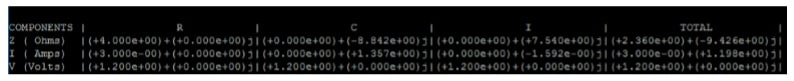CONSTRAINTS

• Pi needs to be to 11 decimal places in your program.
• Formatting needs to be exactly as specified. (Your program will be tested against automated test cases)
• Text to user needs to be easily understandable. You can change the text but the same inputs must be used.
• The program must print your student number, name and the assignment number as specified.
• Types should be used appropriately.
• You must use comments to explain significant lines of code.
• You must use comments to explain how to use the functions and solution